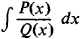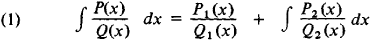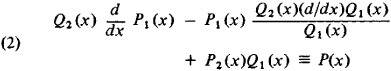The following article is from The Great Soviet Encyclopedia (1979). It might be outdated or ideologically biased.

a method for separating out the rational part of the indefinite inteeralwhere Q(x) is a polynomial of degree n with multiple roots and P(x) is a polynomial of degree mn – 1.

The Ostrogradskii method enables us to write this integral as a sum of two terms, the first of which is a rational function of the variable x, while the second does not contain a rational part. Thuswhere Q1, Q2, P1, and P2 are polynomials of degree n1, n2, m1, and m2, respectively. Moreover, n1 + n2 = n, m1n1–1, m2n2–1, and the polynomial Q2 (x) does not have multiple roots. The polynomial Q1 (x) is the greatest common divisor of the polynomials Q(x) and (d/dx)Q(x), and, consequently, an explicit expression for Q1 (x) can be found by means of, for example, Euclid’s algorithm. Differentiating the right-hand and left-hand sides of equation (1), we obtain the identityThe identity (2) enables us to find an explicit expression for the polynomials P1 (x) and P2 (x) by the method of undetermined coefficients.

The Ostrogradskii method was first put forth in 1844 by M. V. Ostrogradskii.

REFERENCE

Fikhtengol’ts, G. M. Kurs differentsial’nogo i integral’nogo ischisleniia, 7th ed., vol. 2. Moscow, 1969.